Courses

# Chapter 4 - Time Domain Analysis - Notes, Control System, Electrical Engineering Electrical Engineering (EE) Notes | EduRev

## Electrical Engineering (EE) : Chapter 4 - Time Domain Analysis - Notes, Control System, Electrical Engineering Electrical Engineering (EE) Notes | EduRev

The document Chapter 4 - Time Domain Analysis - Notes, Control System, Electrical Engineering Electrical Engineering (EE) Notes | EduRev is a part of the Electrical Engineering (EE) Course Electrical Engineering SSC JE (Technical).
All you need of Electrical Engineering (EE) at this link: Electrical Engineering (EE)

## TIME DOMAIN ANALYSIS

• The time response of a control system is usually divided into tow parts the transient response and the steady state response. Let y(t) denote the time response of a continuous time system. then, in general, is can be written as,

y(t) = yt(t) + yss(t)
where,

yt(t) denotes the transient response and
yss(t) denotes the steady state response.

• In control system, transient response is defined as the time response that goes to zero as time becomes very large. Thus yt(t) has the property.

Lim yt(t) = 0
t → ∝

• The steady-state response is simply that part of the total response that remains after teh transient has died out. Thus the steady-state response can still very in a fixed pattern, such as a sine wave, or a temp function that increases with time.

STANDARD TEST SIGNALS

• The various inputs of disturbances affecting the performance of a system are mathematically represented as standard test signals.
(a) Sudden input: step signal
(b) Velocity Type of Input: Ramp signal
(c) Acceleration type of Input: Parabolic signal
(d) Sudden Shocks: Impulse signal
⇒ signals (a) and (d) are bounded input signals.
⇒ signals (b) and (c) are unbounded input signals.
⇒ Signals (a), (b) and (c) are for time domain analysis.
⇒Signal (d) is important for steady state analysis.
• Every transfer function representing the control system if of particular type and order.
• The steady state analysis depends on the type of the system.
• The type of the system is determined from open loop transfer function G(s)H(s)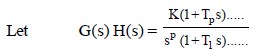If P = 0 ⇒ type-0 system

If P = 1 ⇒ type-1 system

If P = 2 ⇒ type-2 system

......................................

If P = n ⇒ type-n system

• The number of open loop poles occurring at origin determines the type of the system.
• The transient state analysis depends on order of the system.
• The order of the system is obtained from closed loop transfer function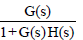• The highest power of [1+G(s) H(s)] determines the order of the system.

• To obtain an expression for error: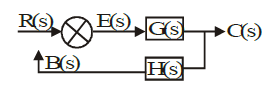E(s) = R(s) - B(s)
E(s) = R(s) - C(s) H(s)
E(s) = R(s) - E(s) G(s) H(s)
E(s) [1 + G(s) H (s)] = R(s)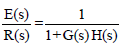Applying the final value theorem,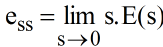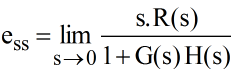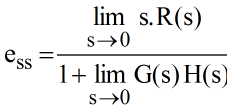Steady State Error for Different types of Inputs

• Step input,

R(s) = A/s

ess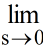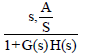ess  =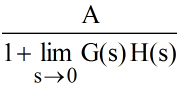K= Position error constant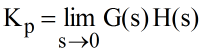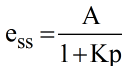• Ramp input.

R(s) = A/s2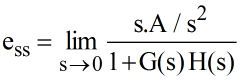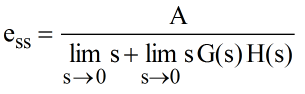Kv = Velocity error constant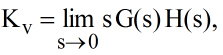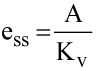• Parabolic input,

R(s) = A/s3

ess =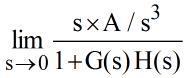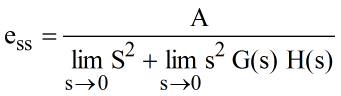Ka = Acceleration error constant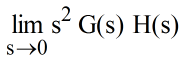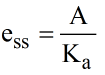Steady State Error for Different Types of Systems
Type-0 system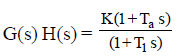• Step input

R(s) = A/s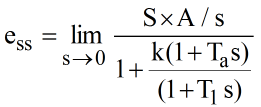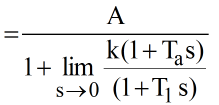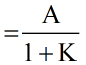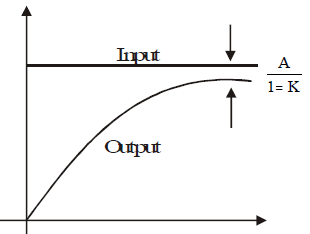• Ramp input,

R(s) = A/s2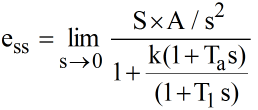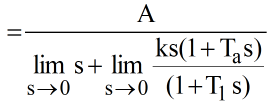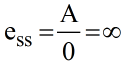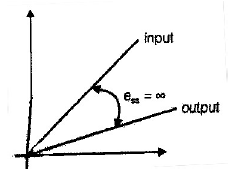• Parabolic input,

R(s) = A/s3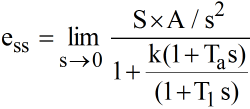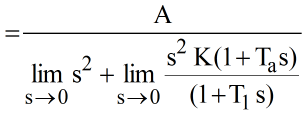=∞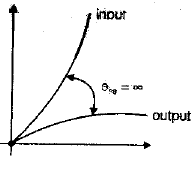Observations
(a) essμ1/K; where K is system gain It means with the in cease of system gain, the value of steady state error will decrease.
(b) The maximum type number of a liner control system is 2. Beyond type 2, the system exhibits non linear behaviour.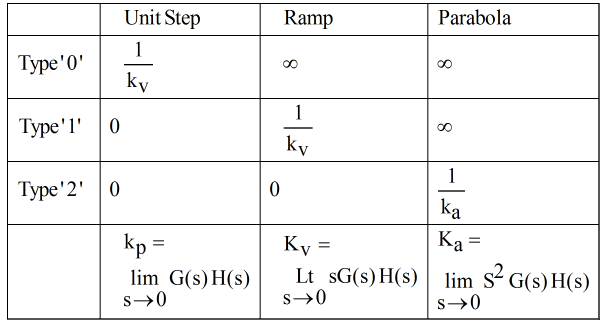TRANSIENT STATE ANALYSIS

• It deals with the nature of response of a system and depends on order of a system.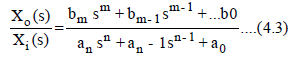Zero Order System

• In equation (4.3) if all the terms except a0, b0 are made 0, then the resulting equation describes a zero order system.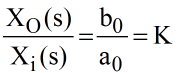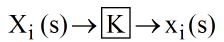• Example: Sensors and transducers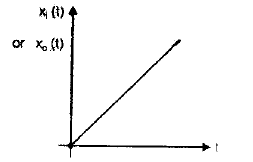• We do not need any time domain analysis because the input, output characteristics are linearly dependent.

First Order System

• In equation (4.3) if all the terms except a1, a0 and b0 are zero then the resulting expression describes a first order system.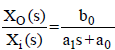Let,

K= gain = b0/a0
T= time constant = a1/a0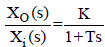• Example: RC-filter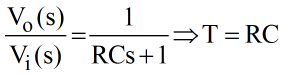• Transient analysis

Let,

Xi(s) = 1/s (unit step)

X0 =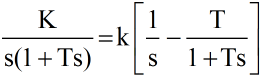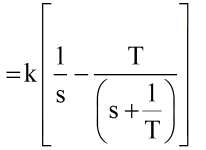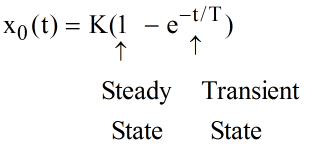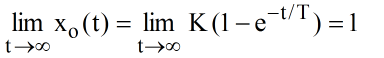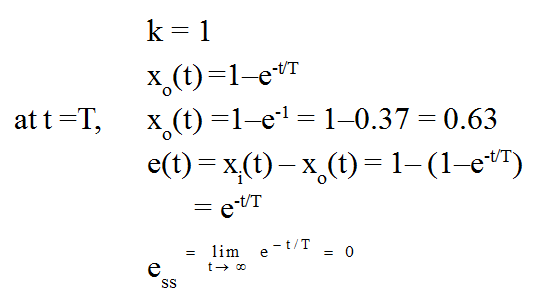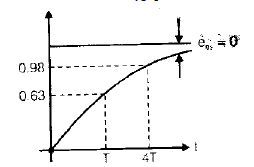• The time constant is defined as time taken by the system response to reach 63% of the final value.
• Example: Thermal system, liquid level system, pneumatic system etc.

Second Order system

• The response of second order system exhibits continuous and sustained oscillations about the steady state value of the input, with the frequency known as undamped natural frequency wn rad/ sec.
• These oscillations are damped to the steady state value of the input using appropriate damping methods:
• The damping is represented as damping factor or damping ratio (x) .
• The standard transfer function of a 2nd order system is therefore expressed in terms of x and wn ; where, wn = undamped natural frequency in rad/sec
• Example: All indicating instruments, RLC network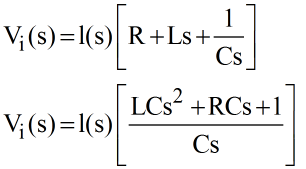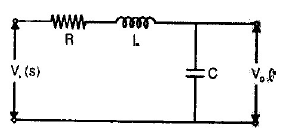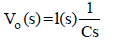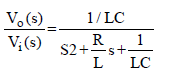Comparing: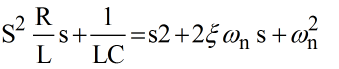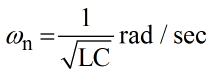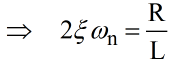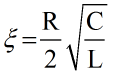• Effect of damping on closed loop poles and nature of response: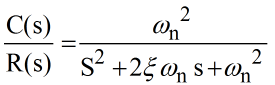Closed loop poles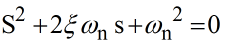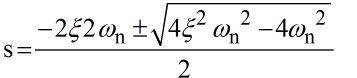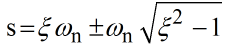Case-1: Undamped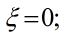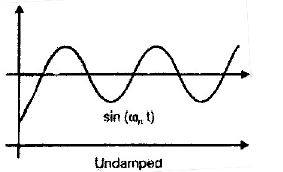case-2: Under damped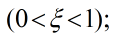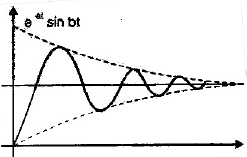case-3: Critical damped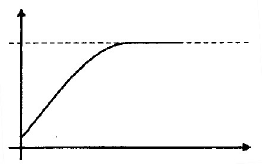case-4: Over damped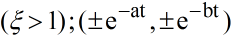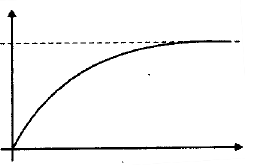• Most of the control system are designed for damping less than 1 because the response is neither too fast ( ξ = 1) nor too slow (ξ >1) in reaching the steady state value of the input.

CHARACTERISTICS OF UNDERDAMPED SYSTEMS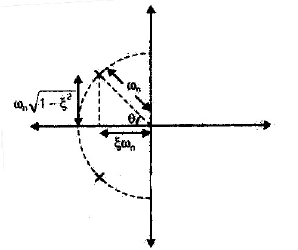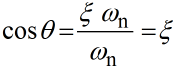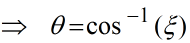(b) Damping coefficient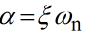(c) Time constant of underdamped response (T)

T=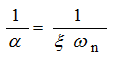(d) Damped natural frequency (ω d)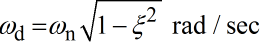TRANSIENT ANALYSIS (UNDERDAMPED RESPONSE)

Let,

R(s) = 1/S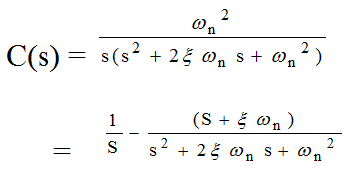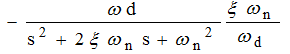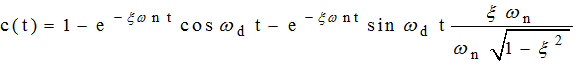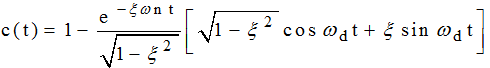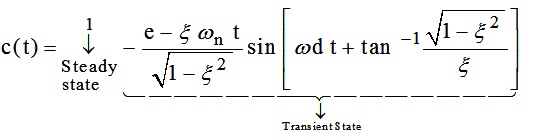• The error is given as,

e(t) = r(t) –c(t)
r(t) = 1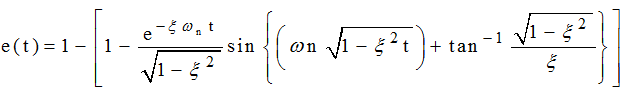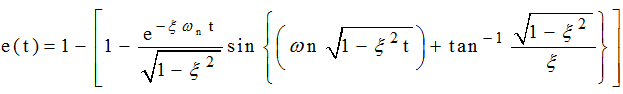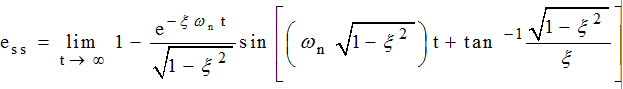• The time response expression given by equation (4.4) indicates that for values of ξ < 1, the response presents exponentially decaying Oscillations having a frequency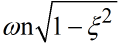and the time constant of exponential decay is 1/ξωn

TIME RESPONSE SPECIFICATION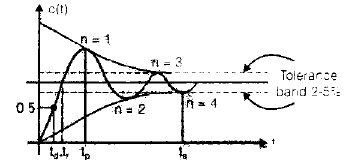Delay Time (td)

• The deley time is the time required for the response to reach half the final value the very first time.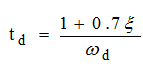Rise Time (tr)

• The rise time is the time required for the response to rise from 10% to 90%, 5% to 95% or 0% to 100% if its final value. For underdamped second order system, the 0% to 100% is normally used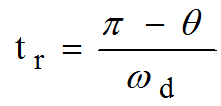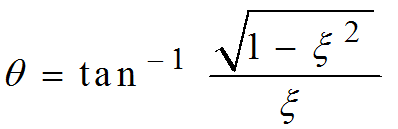peak Time (tp)

• The peak time is the time required for the response to reach the first peak of overshoot.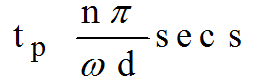For

1st peak, n = 1
2nd peak, n =3

maximum peak Overshoot /maximum percent overshoot

• The maximum overshoot is the maximum peak value of the response curve measured form unity, and is given by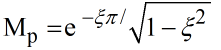• If the final state value of the response differs from unity, then it is common to use the maximum percentage overshoot. It is defined by Maximum

percentave overshoot =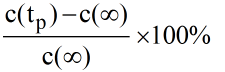• The amount of the maximum percentage overshoot indicates the relative stability of the system.

Settling Time (ts)

• The settling time is the time required for the response curve to reach and stay within a range about the final value of size specified by absolute percentage of the final value (usually 2% or 5%).
• Settling time for the 2% tolerance band - 4T =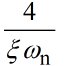• Settling time for the 5% tolerance band = 3T =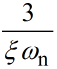TIME RESPONSE ANALTYSIS OF HIGHER ORDER SYSTEMS

• Consider a third order polynomial (Characteristic equation)

1 + G(s) H(s) = 0
s3 + P s2 +q S + K = 0
(s+P1) (s2 + qs + K1)=0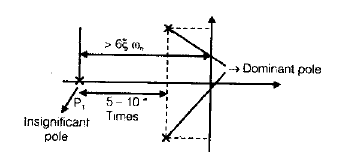• Time response of a higher order system is done by approximating it to second order system with respect to dominant poles. The number of closed loop poles lying in the dominant region must be greater than or equal to number of poles lying in insignificant region

Offer running on EduRev: Apply code STAYHOME200 to get INR 200 off on our premium plan EduRev Infinity!

65 docs|37 tests

,

,

,

,

,

,

,

,

,

,

,

,

,

,

,

,

,

,

,

,

,

,

,

,

,

,

,

;